## Determining the Eigenvalues of a Matrix

Since every linear operator is given by left multiplication by some square matrix, finding the eigenvalues and eigenvectors of a linear operator is equivalent to finding the eigenvalues and eigenvectors of the associated square matrix; this is the terminology that will be followed. Furthermore, since eigenvalues and eigenvectors make sense only for square matrices, throughout this section all matrices are assumed to be square.

Given a square matrix A, the condition that characterizes an eigenvalue, λ, is the existence of a nonzero vector x such that A x = λ x; this equation can be rewritten as follows: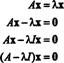This final form of the equation makes it clear that x is the solution of a square, homogeneous system. If nonzero solutions are desired, then the determinant of the coefficient matrix—which in this case is A − λ I—must be zero; if not, then the system possesses only the trivial solution x = 0. Since eigenvectors are, by definition, nonzero, in order for x to be an eigenvector of a matrix A, λ must be chosen so that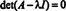When the determinant of A − λ I is written out, the resulting expression is a monic polynomial in λ. [A monic polynomial is one in which the coefficient of the leading (the highest‐degree) term is 1.] It is called the characteristic polynomial of A and will be of degree n if A is n x n. The zeros of the characteristic polynomial of A—that is, the solutions of the characteristic equation, det( A − λ I) = 0—are the eigenvalues of A.

Example 1: Determine the eigenvalues of the matrix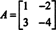First, form the matrix A − λ Ia result which follows by simply subtracting λ from each of the entries on the main diagonal. Now, take the determinant of A − λ I:This is the characteristic polynomial of A, and the solutions of the characteristic equation, det( A − λ I) = 0, are the eigenvalues of A:In some texts, the characteristic polynomial of A is written det (λ I − A), rather than det ( A − λ I). For matrices of even dimension, these polynomials are precisely the same, while for square matrices of odd dimension, these polynomials are additive inverses. The distinction is merely cosmetic, becaues the solutions of det (λ I − A) = 0 are precisely the same as the solutions of det ( A − λ I) = 0. Therefore, whether you write the characteristic polynomial of A as det(λ I − A) or as det( A − λ I) will have no effect on the determination of the eigenvalues or their corresponding eigenvectors.

Example 2: Find the eigenvalues of the 3 by 3 checkerboard matrixThe determinant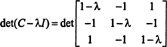is evaluated by first adding the second row to the third and then performing a Laplace expansion by the first column: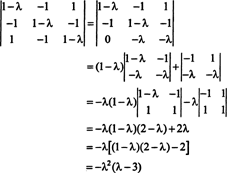The roots of the characteristic equation, −λ 2(λ − 3) = 0, are λ = 0 and λ = 3; these are the eigenvalues of C.